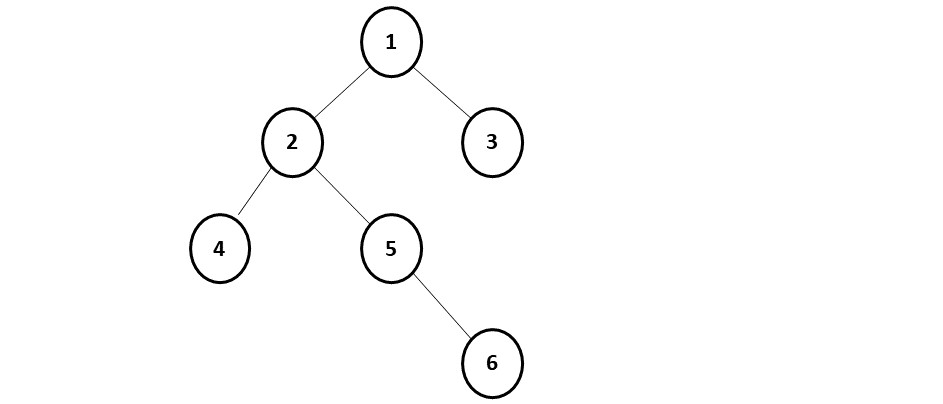# Morris Inorder Traversal of a Binary tree

Problem Statement: Write a program for Morris Inorder Traversal of a Binary Tree.

Example:

```Input:Output: Inorder Traversal of this binary tree will be:- 4,2,5,6,1,3
```

Disclaimer: Don’t jump directly to the solution, try it out yourself first.

### Solution:

Intuition: We first need to understand what we do in inorder traversal: Left, Current, Right.

If we draw a path of our traversal, we will get:This is exactly our threaded binary tree, which we use in the algorithm.

We need to figure out how we can go back in the tree from a child to a parent without using recursion. We break this tree into small sub-trees (marked in red):In figure (i), we see that when we are at node 4 and this red subtree has no right child, we move to the parent of this subtree, i.e node 2. Similarly, in figure(ii), when we are at node 6, this red subtree has no right child, therefore we move to its parent, i.e node 1.

We observe a pattern that whenever we are at the last node of a subtree such that the right child is pointing to none, we move to the parent of this subtree.

Approach:

When we are currently at a node, the following cases can arise:

• Case 1: When the current node has no left subtree. In this scenario, there is nothing to be traversed on the left side, so we simply print the value of the current node and move to the right of the current node.
• Case 2: When there is a left subtree and the right-most child of this left subtree is pointing to null. In this case we need to set the right-most child to point to the current node, instead of NULL and move to the left of the current node.
• Case 3: When there is a left subtree and the right-most child of this left-subtree is already pointing to the current node. In this case we know that the left subtree is already visited so we need to print the value of the current node and move to the right of the current node.

Note: Case 3 is very important as we need to remove the new links added to restore the original tree.

To summarize, at a node whether we have to move left or right is determined whether the node has a left subtree. If it doesn’t we move to the right. If there is a left subtree then we see its rightmost child. If the rightmost child is pointing to NULL, we move the current node to its left. If the rightmost child is already pointing towards the current node, we remove that link and move to the right of the current node. We will stop the execution when the current points to null and we have traversed the whole tree.

Code:

## C++ Code

``````#include <bits/stdc++.h>

using namespace std;

struct node {
int data;
struct node * left, * right;
};

vector < int > inorderTraversal(node * root) {
vector < int > inorder;

node * cur = root;
while (cur != NULL) {
if (cur -> left == NULL) {
inorder.push_back(cur -> data);
cur = cur -> right;
} else {
node * prev = cur -> left;
while (prev -> right != NULL && prev -> right != cur) {
prev = prev -> right;
}

if (prev -> right == NULL) {
prev -> right = cur;
cur = cur -> left;
} else {
prev -> right = NULL;
inorder.push_back(cur -> data);
cur = cur -> right;
}
}
}
return inorder;
}

struct node * newNode(int data) {
struct node * node = (struct node * ) malloc(sizeof(struct node));
node -> data = data;
node -> left = NULL;
node -> right = NULL;

return (node);
}

int main() {

struct node * root = newNode(1);
root -> left = newNode(2);
root -> right = newNode(3);
root -> left -> left = newNode(4);
root -> left -> right = newNode(5);
root -> left -> right -> right = newNode(6);

vector < int > inorder;
inorder = inorderTraversal(root);

cout << "The Inorder Traversal is: ";
for (int i = 0; i < inorder.size(); i++) {
cout << inorder[i] << " ";
}

return 0;
}
``````

Output: The Inorder Traversal is: 4 2 5 6 1 3

Time Complexity: O(N).

Space Complexity: O(1)

Reason: We are not using recursion.

## Java Code

``````class Solution {
public List<Integer> inorderTraversal(TreeNode root) {
List<Integer> inorder = new ArrayList<Integer>();

TreeNode cur = root;
while(cur != null) {
if(cur.left == null) {
cur = cur.right;
}
else {
TreeNode prev = cur.left;
while(prev.right != null && prev.right != cur) {
prev = prev.right;
}

if(prev.right == null) {
prev.right = cur;
cur = cur.left;
}
else {
prev.right = null;
cur = cur.right;
}
}
}
return inorder;
}
}``````

Time Complexity: O(N).

Space Complexity: O(1)

Reason: We are not using recursion.